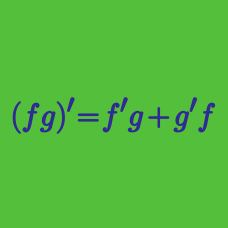Calculus

# Chain Rule

What is the derivative of $y=4\tan^{4} x?$

$f(x)$ is a differentiable function such that $f(2)=3$ and $f'(2)=3.$ If $g(x)=\left(x^2f(x)\right)^2,$ what is the value of $g'(2)?$

What is the derivative of $y=3 ^{x^ 6-4}?$

Consider the function $f(x)=\log_{8} \left[ (3x-1)^3 \right] .$ What is the value of $a$ such that $f'(a)=\frac{9}{\ln 8}?$

If the instantaneous rate of change of $y=\sin^{-1} (3x)$ at $x=\frac{1}{6}$ can be expressed as $\frac{18}{\sqrt{a}},$ what is the value of $a?$

×